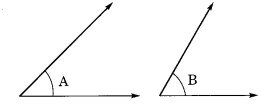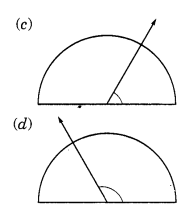# NCERT Solutions For Class 6 Maths Understanding Elementary Shapes Ex 5.4

ncert textbook

## NCERT Solutions For Class 6 Maths Understanding Elementary Shapes Ex 5.4

NCERT Solutions For Class 6 Maths Chapter 5 Understanding Elementary Shapes Ex 5.4

Exercise 5.4

Question 1.
What is the measure of (i) a right angle (ii) a straight angle?
Solution:
(i) Measure of a right angle = 90°
(ii) Measure of a straight angle = 180°

Question 2.
Say True or False:
(a) The measure of an acute angle < 90°
(b) The measure of an obtuse angle < 90°
(c) The measure of a reflex angle > 180°
(d) The measure of one complete revolution = 360°
(e) If m ∠A = 53° and ∠B = 35°, then m∠A > m∠B.
Solution:
(a) True
(b) False
(c) True
(d) True
(e) True

Question 3.
Write down the measures of
(a) some acute angles
(b) some obtuse angles
Solution:
(a) 25°, 63° and 72° are acute angles.
(b) 105°, 120° and 135° are obtuse angles.

Question 4.
Measure the angles given below using the protractor and write down the measure.Solution:
(a) 45°
(b) 125°
(c) 90°
(d) ∠1 = 60°, ∠2 = 90°, ∠3 = 125°

Question 5.
Which angle has a large measure? First estimate and then measure.
Measure of Angle A =
Measure of Angle B =Solution:
Measure of Angle A = 40°
Measure of Angle B = 60°.

Question 6.
From these two angles which has large measure? Estimate and then confirm by measuring them.
Solution:
The opening of angle (b) is more than angle (a).∴ Measure of angle (a) = 45°
and the measure of angle (b) = 60°

Question 7.
Fill in the blanks with acute, obtuse, right or straight:
(a) An angle whose measure is less than that of a right angle is ……… .
(b) An angle whose measure is greater than that of a right angle is ……… .
(c) An angle whose measure is the sum of the measures of two right angles is ……… .
(d) When the sum of the measures of two angles is that of a right angle, then each one of them is ……… .
(e) When the sum of the measures of two angles is that of a straight angle and if one of them is acute then the other should be ……… .
Solution:
(a) acute
(b) obtuse
(c) straight
(d) acute
(e) obtuse

Question 8.
Find the measure of the angle shown in each figure. (First estimate with your eyes and than find the actual measure with a protractor).Solution:
(a) Measure of the angle = 40°
(b) Measure of the angle = 130°
(c) Measure of the angle = 65°
(d) Measure of the angle = 135°.

Question 9.
Find the angle measure between the hands of the clock in each figure:Solution:
(i) The angle between hour hand and minute hand of a clock at 9.00 a.m = 90°
(ii) The angle between the hour hand and minute hand of a clock at 1.00 p.m = 30°
(iii) The angle between the hour hand and minute hand of a clock at 6.00 p.m = 180°.

Question 10.
Investigate: In the given figure, the angle measures 30°. Look at the same figure through a magnifying glass. Does the angle becomes larger? Does the size of the angle change?Solution:
It is an activity. So try it yourself.

Question 11.
Measure and classify each angle:Angle Measure Type ∠AOB ∠AOC ∠BOC ∠DOC ∠DOA ∠DOB

Solution:

 Angle Measure Type ∠AOB 40° Acute angle ∠AOC 125° Obtuse angle ∠BOC 85° Acute angle ∠DOC 95° Obtuse angle ∠DOA 140° Obtuse angle ∠DOB 180° Straight angle